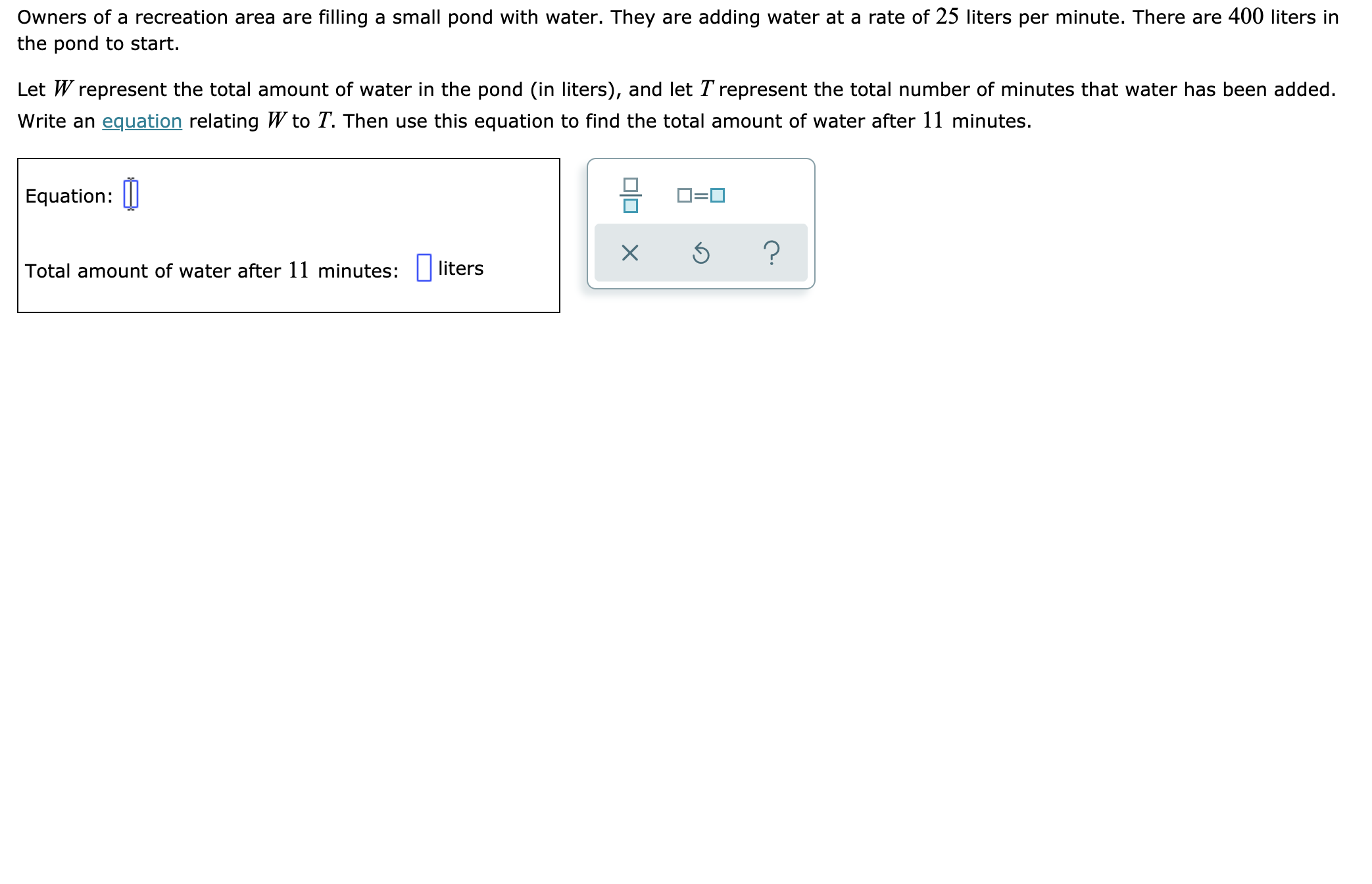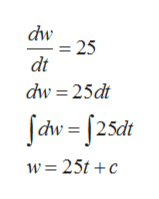Owners of a recreation area are filling a small pond with water. They are adding water at a rate of 25 liters per minute. There are 400 liters inthe pond to start.Let W represent the total amount of water in the pond (in liters), and let T represent the total number of minutes that water has been added.Write an equation relating W to T. Then use this equation to find the total amount of water after 11 minutes.Equation:?Total amount of water after 11 minutes: liters

Question

See attachedhelp_outlineImage TranscriptioncloseOwners of a recreation area are filling a small pond with water. They are adding water at a rate of 25 liters per minute. There are 400 liters in the pond to start. Let W represent the total amount of water in the pond (in liters), and let T represent the total number of minutes that water has been added. Write an equation relating W to T. Then use this equation to find the total amount of water after 11 minutes. Equation: ? Total amount of water after 11 minutes: liters fullscreen
Step 1

Given that

Rate of filling of water to the pond is 25

Step 2

The rate of filling of water to the pond ishelp_outlineImage Transcriptionclosedw = 25 dt dw 25dt fdw=f25dt w 25tc fullscreen
Step 3

At t = 0, W ...

Want to see the full answer?

See Solution

Want to see this answer and more?

Our solutions are written by experts, many with advanced degrees, and available 24/7

See Solution
Tagged in

Calculus